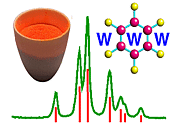Intensity of Diffraction: the Structure Factor Equation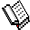Course Material Index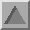Section Index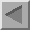Previous Page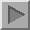Next Page

Intensity of Diffraction: the Structure Factor Equation

If the previous two sections answer the question when?, this section starts to address the question how much? The concepts of Ewald sphere and reciprocal lattice or Bragg's law predict when diffraction will occur but give no indication of the intensity of a given diffraction event.

 F(S)  = Σn fne2π i S.rn
We describe the position, r, of atoms by the use of fractional coordinates (x, y, z) of the repeat units a, b, c of the crystal, i.e.
 r = xa +  yb + zc
and substituting this gives:
 F(S)  = Σn fne2π i S.(xa + yb + zc ) = Σn fne2π i (S.ax + S.by + S.cz)
But we know that for a large number, N, of repeats the 3 von Laue conditions will apply. So writing these (S.a = h, S.b = k, S.c = l) into the above equation gives:
 F(S)  = Σn fne2π i (hx + ky + lz )
This effectively is the final answer required except to note that sometimes the summation is written in the form:
 F(S)  = Σx Σy Σz fne2π i (hx + ky + lz )
thus recognising that to sum over all space (x, y, z) within a unit cell is equivalent in diffraction terms to summing over all atoms (n) within the unit cell.

If Bragg's equation is the most well-known equation, then the structure factor equation must be the second most well-known. As much of crystallography is involved with determining atomic structure from the intensity of diffraction spots/peaks, then this equation acts as the basic computational link between the two. At first it might appear to be quite complex given the summations and use of complex numbers. However this is not so; even if students cannot understand its derivation, the Structure Factor equation is relatively easy to use as we will show later.Course Material IndexSection IndexPrevious PageNext Page
 © Copyright 1997-2006.  Birkbeck College, University of London. Author(s): Paul Barnes Martin Vickers